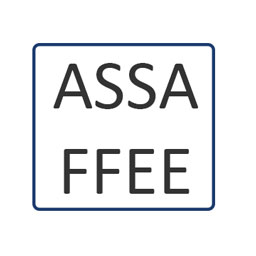### D - Double letters

##### Languages: C, C++, Java, Python, ... (details)

You are given a $4$-character string $S$ consisting of uppercase English letters. Determine if $S$ consists of exactly two kinds of characters which both appear twice in $S$.#### Input

The input contains one line with the string $S$ ($|S|=4$).

#### Output

If $S$ consists of exactly two kinds of characters which both appear twice in $S$ print "Yes", otherwise print "No" (without the quotes).

Input
ASSA
Output
Yes
Input
STOP
Output
No
Input
FFEE
Output
Yes
Input
FREE
Output
No